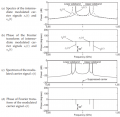# how carrier be suspressed in DSB modulation?

#### fansaiti

Joined Apr 1, 2022
6
in books, AM always described as y(t) = [A + m(t)].c(t), where c(t) refers to carrier wave, m(t) is baseband/modulating signal.
A sometimes be explained as the amplitude of m(t). Because of the existance of 'A', the carrier part be kept together with upper and lower side band.
However, when we decribe DSB, 'A' be removed from equation, as a result, carrier be suspressed and only upper and lower side band remains.
I am not clear, how does 'A' be represented in hardware(real circuits)? How to remove 'A' in circuits?

#### Papabravo

Joined Feb 24, 2006
18,793
Careful design of the mixer can eliminate the carrier and the modulating signal, leaving only the two sidebands. Alternatively, the carrier can be removed with a filter that suppresses the carrier. The basic operation of the mixer involves the multiplication of sinusoids which gives rise to the sidebands.

•fansaiti

#### crutschow

Joined Mar 14, 2008
30,102
An analog multiplier with two inputs consisting of the carrier and the modulating signal will generate a DSB signal with no carrier.
(If the modulating signal is zero, then you are multiplying zero times the carrier signal which is zero at the output).

•fansaiti

#### fansaiti

Joined Apr 1, 2022
6
Careful design of the mixer can eliminate the carrier and the modulating signal, leaving only the two sidebands. Alternatively, the carrier can be removed with a filter that suppresses the carrier. The basic operation of the mixer involves the multiplication of sinusoids which gives rise to the sidebands.
Great and thanks a lot^_^ In other words, the 'A' is anyway exist inside a baseband/modulating signal, but if we wanna a DSB signal, a filter circuit should be introduced to kill the carrier part ? Actually, some books said, 'A' is a DC signal which is added before multiplication, as result AM with carrier produced. When you want to produce a DSB, just remove this DC signal inputting in your circuit. It seems that, theoretically, there are two methods to produce a DSB signal, 1. produce a AM first then suspress the carrier by filter; 2. multiply the baseband/modulating signal to carrier directly, without adding DC('A') signal.
which method is applied in real ampitude modulate circuits ?

#### Ramussons

Joined May 3, 2013
1,273
A Double Balanced Modulator, like the MC 1496, does the job well.

#### fansaiti

Joined Apr 1, 2022
6
An analog multiplier with two inputs consisting of the carrier and the modulating signal will generate a DSB signal with no carrier.
(If the modulating signal is zero, then you are multiplying zero times the carrier signal which is zero at the output).
Thanks. How do you think modulating signal ? [A+m(t)] or just m(t) ? I prefer to think just m(t) as modulating signal, while A is a DC be added artifically when designing circuit.

#### fansaiti

Joined Apr 1, 2022
6
Mr zapper, could you please explain ' If the modulating signal is zero' ?

#### crutschow

Joined Mar 14, 2008
30,102
Mr zapper, could you please explain 'If the modulating signal is zero' ?
You are multiplying two signals together.
If one is zero volts than the output is also zero.
So if the modulating signal is zero volts, then there is no signal, and thus no carrier present at the output.
That's how the carrier is suppressed.

But note that, at the receiver, the carrier must be recreated to properly demodulate the signal.
Sometimes a small value of carrier signal (pilot carrier) is transmitted to make it easier for the receiver generate the carrier for the demodulation.

Also, since the two sidebands have duplicate (and redundant) information, one of the sidebands can be eliminated (by filtering or other techniques) to generate the more commonly used single-sideband signal.
That reduces the required RF bandwidth and allows concentration of all the transmitted power into one sideband.

#### fansaiti

Joined Apr 1, 2022
6
An analog multiplier with two inputs consisting of the carrier and the modulating signal will generate a DSB signal with no carrier.
(If the modulating signal is zero, then you are multiplying zero times the carrier signal which is zero at the output).
Mr zapper, could you please explain ' If the modulating signal is zero ' ?
Thanks. How do you think modulating signal ? [A+m(t)] or just m(t) ? I prefer to think just m(t) as modulating signal, while A is a DC be added artifically when designing circuit.
You are multiplying two signals together.
If one is zero volts than the output is also zero.
So if the modulating signal is zero volts, then there is no signal, and thus no carrier present at the output.
That's how the carrier is suppressed.

But note that, at the receiver, the carrier must be recreated to properly demodulate the signal.
Sometimes a small value of carrier signal (pilot carrier) is transmitted to make it easier for the receiver generate the carrier for the demodulation.

Also, since the two sidebands have duplicate (and redundant) information, one of the sidebands can be eliminated (by filtering or other techniques) to generate the more commonly used single-sideband signal.
That reduces the required RF bandwidth and allows concentration of all the transmitted power into one sideband.
Take this equation as example: y(t) = [A + m(t)].c(t), where c(t) refers to carrier wave, m(t) is baseband/modulating signal,
A as the amplitude of m(t). The modulating signal includes A and m(t), let A=0, we obtain a DSB(carrier suspressed), while let A>0, we obtain a AM with carrier and double side band. Do you agree? If it is, do you know, how should A be introduced into real circuit? By adding a DC over m(t) signal ?

#### nsaspook

Joined Aug 27, 2009
9,987
There are the traditional analog filter methods and newer digital methods of SSB/DSB-SC modulation.•